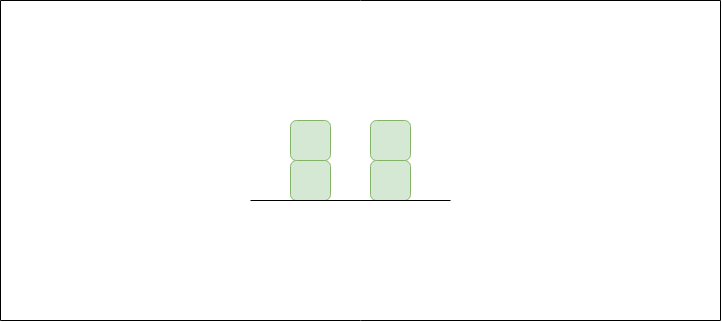Given n non-negative integers representing an elevation map where the width of each bar is 1, compute how much water it is able to trap after raining.

Examples:

``````Input: arr[]   = {2, 0, 2}
Output: 2
Explanation:
The structure is like below
````````````We can trap 2 units of water in the middle gap.
``````

METHOD 1

``````import org.junit.Test;

import static org.junit.Assert.assertEquals;

/**
* Question   : Trapping rain water
* Complexity : Time: O(n) ; Space: O(1)
* Time       : 2020/06/10
* Author     : Max
*/
public class TrappingRainWater {
static int maxWater(int[] arr) {
if (arr.length == 0) {
return 0;
}

int n = arr.length;

int leftMax = Integer.MIN_VALUE, rightMax = Integer.MIN_VALUE;
int l = 0, h = n - 1;
int trap = 0;

while (l < h) {
if (arr[l] < arr[h]) {
if (arr[l] > leftMax) {
leftMax = arr[l];
} else {
trap += leftMax - arr[l];
}
l++;
} else {
if (arr[h] > rightMax) {
rightMax = arr[l];
} else {
trap += rightMax - arr[h];
}
h--;
}
}

return trap;
}

@Test
public void test() {
assertEquals(2, maxWater(new int[]{2, 0, 2}));
assertEquals(7, maxWater(new int[]{3, 0, 2, 0, 4}));
assertEquals(6, maxWater(new int[]{0, 1, 0, 2, 1, 0, 1, 3, 2, 1, 2, 1}));
}
}
``````